# Line Graph Worksheets

Line graph worksheets have ample practice skills to analyze, interpret and compare the data from the graphs. Exercises to draw line graphs and double line graphs with a suitable scale; labeling the axes; giving a title for the graph and more are included in these worksheets.

Interpreting Line Graph: Easy

Line graph worksheets have three interesting scenarios with graphs given. Read the line graph and answer the word problems in each worksheet.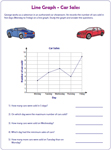Interpreting Line Graph: Moderate

Line graphs on different themes are shown in these worksheets. Interpret the data from the line graph and answer the questions.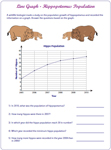Interpreting Line Graph: Difficult

Line graphs in these worksheets represent more than ten data. Read and interpret the graph carefully to answer the questions.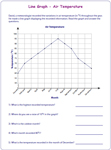Drawing Line Graph: Easy

In these worksheets, data for which the graph to be represented are given. Read the data, plot points and draw lines to complete the graph.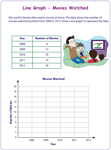Drawing Line Graph: Moderate

The number usage (given data) gradually increases in this level. Plot points on the graph to represent the data and join them to make a line graph.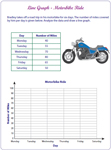Drawing Line Graph: Difficult

Numerous data are used in these worksheets. Attentively read the data and represent it on the grid to draw the line graph.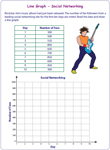Title, Scale, Labeling Axis, and Graphing

Draw a line graph for the represented data. Make an appropriate scale, label the x axis, y axis and write your own title for the graph.In these worksheets two sets of data are compared. Both the data are represented as a double line graph. Read them and answer the questions.Drawing Double Line Graph

Two sets of data are given. Analyze the data, make a suitable scale and draw double line graph. Label the axes and give a suitable title for the graph.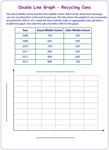The entire collection of line graph worksheets can be downloaded in a jiffy!

Related Worksheets